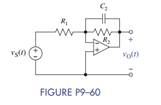### Create an Account

Home / Questions / For the inverting OP AMP circuit shown in Figure P9–60 write a differential equation for v...

# For the inverting OP AMP circuit shown in Figure P9–60 write a differential equation for vO t in terms of the elements and vS t Assume

For the inverting OP AMP circuit shown in Figure P9–60, write a differential equation for vO(t) in terms of the elements and vS(t). Assume vC (0) = 0. Then let vS(t) = e−10t u (t) V, R1 =1kΩ, R2 = 10 kΩ, and C = 1 μF and using Laplace techniques, find the output vO(t).Jun 15 2020 View more View LessSubscribe To Get Solution